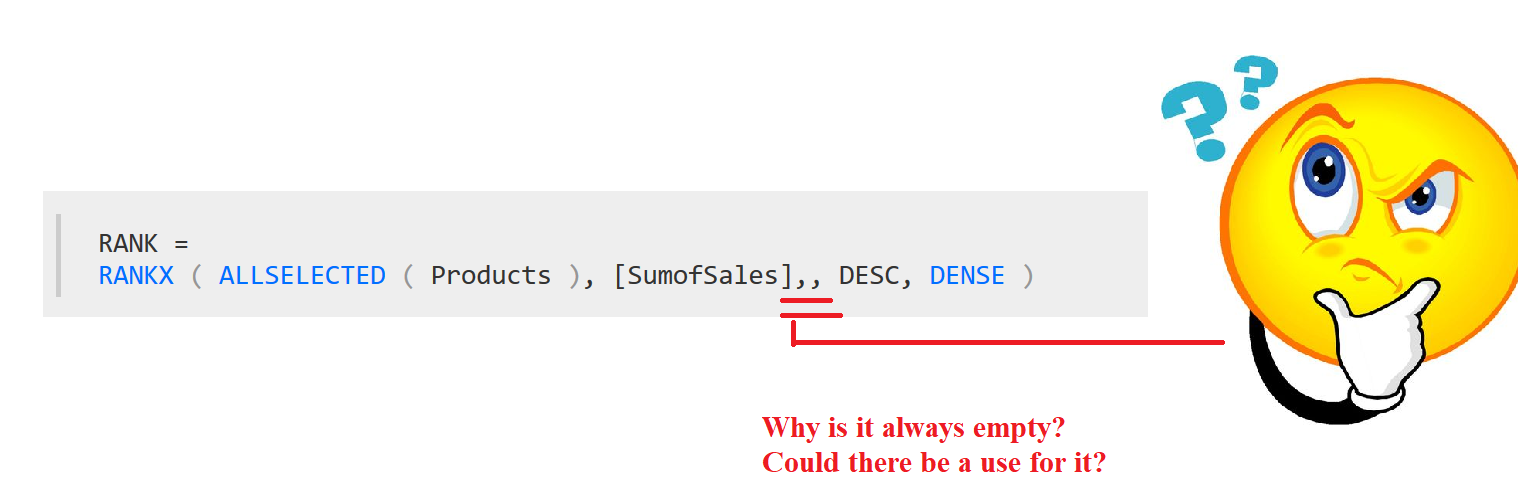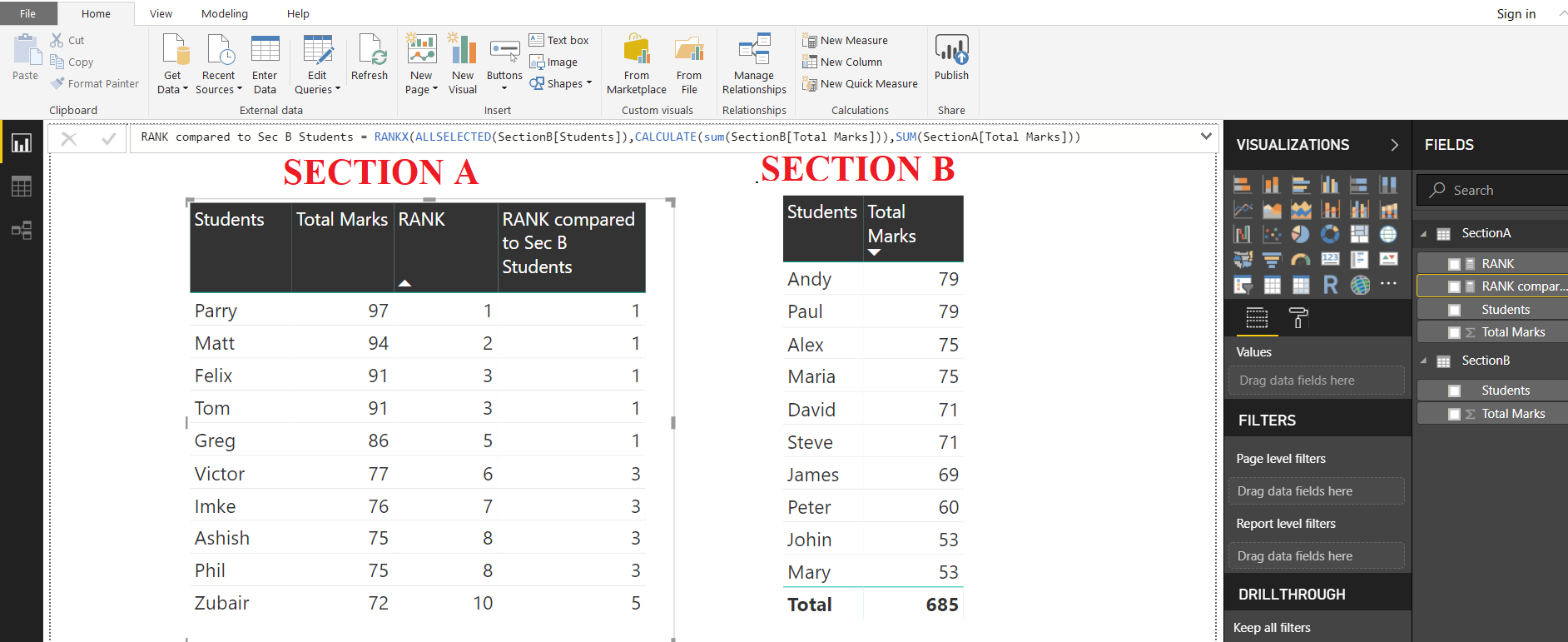# Using VALUE parameter in RANKX

Many of us who have used this function have rarely if ever seen the VALUE argument of RANKX function in use.This post explores a very interesting use of this “VALUE” parameter

BUT FIRST …LETS RECALL THE BASICS

Here is the link to official Microsoft documentation

RANKX takes the following parameters out of which only first 2 are mandatory

`RANKX(Table,Expression,Value,Order,Ties)`

“Expression” in above formula is evaluated for each row of table argument and builds a list of numbers
“Value” is the ScalarValue whose RANK is to be found

So why do we omit it… The reason being

“When the value parameter is omitted, the value of expression at the current row is automatically used by the DAX Engine”
And this makes perfect sense because more often than not we are determining the RANK of a number within a list containing that number”

However, This leads us to a very interesting use of this function

“We can use an entirely different table to RANK a particular value

Lets take an Example. Following are the Scores of 2 sections of Grade 8 in a SchoolTo get the RANK of Section A students within their own section we can use a simple DAX measure

```RANK = RANKX ( ALLSELECTED ( SectionA[Students] ), CALCULATE ( SUM ( SectionA[Total Marks] ) ) )```But how about the PERFORMANCE of Section A students compared to PERFORMANCE OF Section B Students
This is where “VALUE” parameter will come in Handy

The MEASURE to be used is

```RANK compared to Sec B Students = RANKX ( ALLSELECTED ( SectionB[Students] ), CALCULATE ( SUM ( SectionB[Total Marks] ) ), SUM ( SectionA[Total Marks] ) )```

The resultsSo the top 5 students in Section A (Parry, Matt, Felix, Tom, Greg) have better scores than Top Ranked Student in Section B

Interesting use…Isn’t it

What do you think?